Home | Study Links | Rhymes |

## Square the Circle - 396 x 8 = 3168

Squaring the circle means constructing a square with the same area as that of a given circle
or visa versa - using only a pair of compasses for drawing circles and a ruler for drawing
straight lines. Strive for accuracy.

I began experimenting by using one measurement, 396 millimetres, the diameter of one circle.
The numbers 3 6 9 and 3168 are interesting and I began including them in my calculations.

Of course, now that the technique is known, any circle diameter will do.
The square area in my experiment is (a-b 2772 mm x 2) ² = 30735936.
My circle radius result was 3127.8670511888 and an area of 30735936.0000007…
It has an accuracy of 99.999999999997 %. I have not found anything better.

My drawing program is not the best and it maxed out.
I suspect that by using a better drawing program the accuracy can be improved even more.
(In drawing programs, circle perimeters come in segments and must be taken into account.)
Perfect circles are required.) Please let me know your results, should you try this.

Diagram 1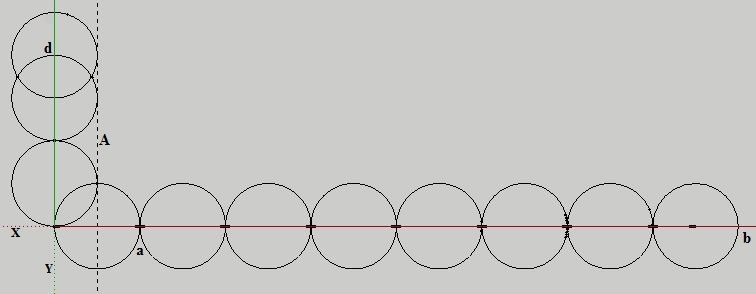1. place 8 circles along the X-axis and 2.5 on the Y-axis.

Diagram 2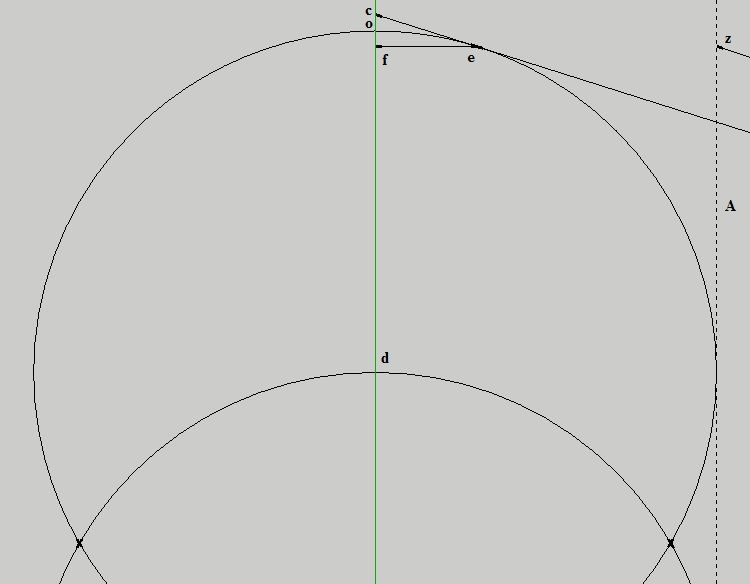3. Connect a line from b (diagram 1) to c (on the Y-axis), touching the circle at e as accurate
as possible. (This step is important!)
4. To this b-c line, and circle connection point e, add radius d-e.
5. Add line e-f, it makes the number 7 shape.
(I did not use degrees, I only indicated what they are as found.)

Diagram 3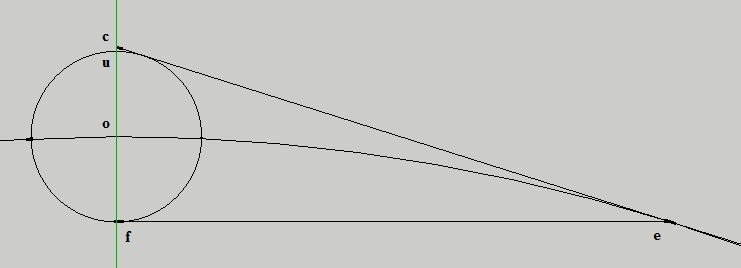6. Draw outher circle, begin with radius o-f.
7. Guide line B is a paralel line to e-d from diagram 2.
8. Zoom in to the 3 small circles.

Diagram 4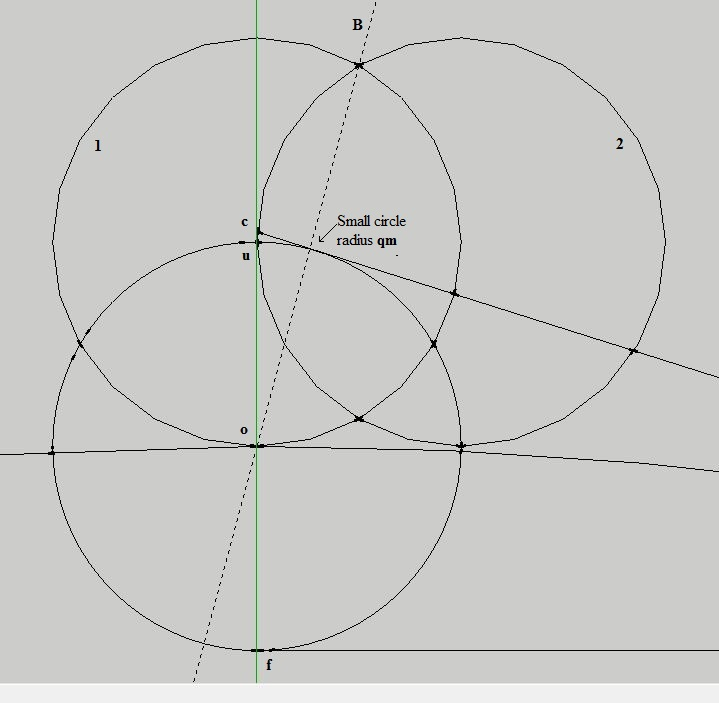9. See diagram 5 for information before returning here.
11. Circle radius l-m. This circle connects to and sits above the small circle.
12. Draw guide line C, from o (diagram 3) to q (connect accurately with q).
13. Draw circle radius h-p giving point n and the diameter for the inner circle.
14. Draw circle radius o-n (diagram 3), giving point v and guide line E.

Diagram 5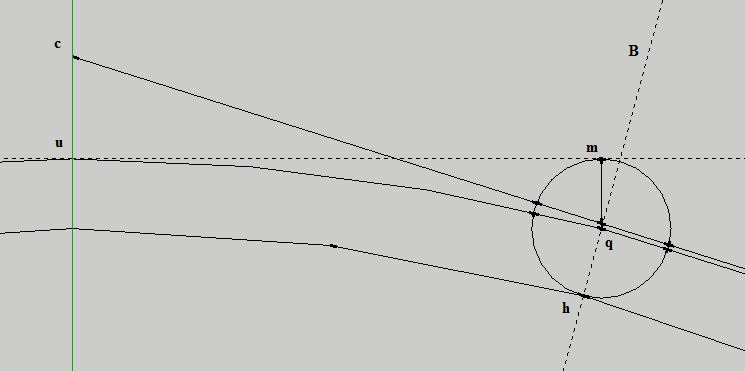10. Circle radius j-i on the B guide line. This small circle fills the narrow "gap".

Diagram 6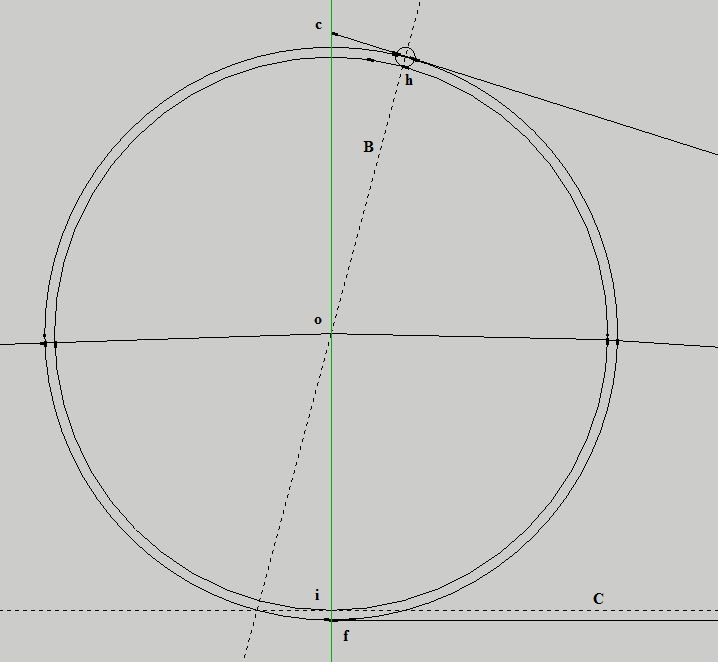14. Draw guide line E through v (diagram 3) to guide line A, making intersection point z.

Diagram 7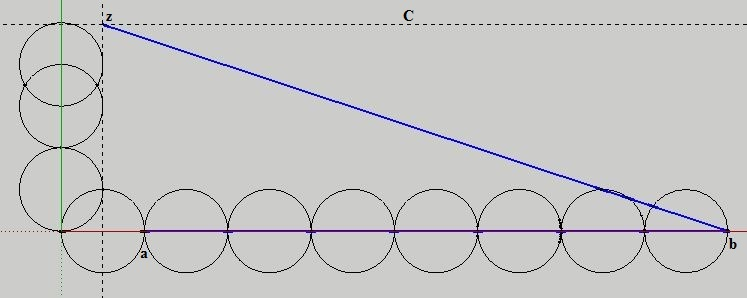15. Draw line b to z gives the circle radius.
16. Circle area (radius b-z) ² x π should closely match Square area (line a-b x 2) ²